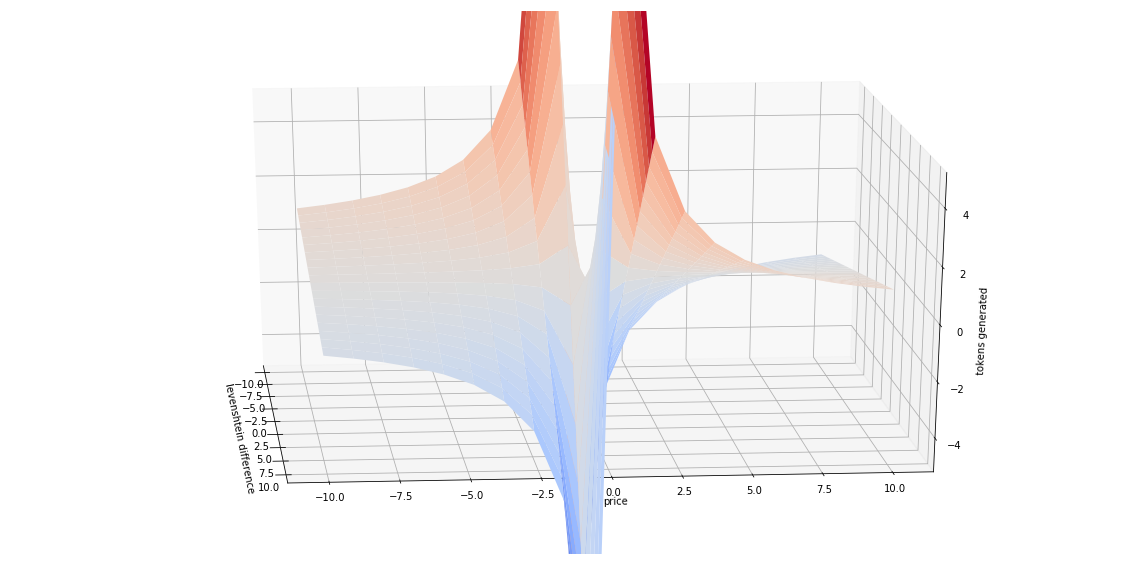Token generation

· 8 mins read

Implementing a Levenshtein based token generation

Introduction

While working on the SDG market one of the first steps was reviewing the token generation mechanisms. A simple character count was implemented on the PHP api. Which proved a good opportunity for me to demonstrate the power of a python based simple command line tool. I started implementing it by writing the Levenshtein function from scratch. This quickly proved inefficient since the Levenshtein function has a time complexity of $O(n^2)$. The logical next step was to use an existing implementation. There seemed to be a pretty well optimized C implementation which led to the API executing better although the execution time was still unreasonably long.

My initial conclusion was that the Levenshtein function had no hope given its huge complexity. I also figured that’s why it wasn’t implemented on the web app which we outsourced. This prompted me to further reflect on how we could make it a prime goal of ours that people can easily implement their token generation functions through a flexible and lightweight API we could give them. The reflection regarding that is still not complete but in the meantime, I found a solution to our Levenshtein problem.

def compute_levenshtein(a, b):
size_x = len(a)
size_y = len(b)

matrix = np.zeros((size_x, size_y))
for x in range(size_x):
matrix[x, 0] = x
for y in range(size_y):
matrix[0,y] = y

for x in range(1, size_x):
for y in range(1, size_y):
if a[x-1] == b[y-1]:
matrix[x, y] = min(
matrix[x-1, y]+1,
matrix[x-1, y-1],
matrix[x, y-1]+1
)
else:
matrix[x, y] = min(
matrix[x-1, y],
matrix[x-1, y-1],
matrix[x, y-1]+1
)
return matrix[size_x-1, size_y-1]

This method although theoretically correct simply wasn’t ideal for our use case since we were diffing whole repositories and the diff strings were often times simply too large. This often led to memory errors. The first step was to use the python-Levenshtein API. That got rid of the memory errors but still had unreasonable execution time. The solution was therefore to parallelize the implementation. Granted python might not be the ideal language for parallel processing the threading library proved sufficient for us; leading large repositories to parse almost instantly instead of taking several minutes with the blocking implementation. Here is the current algorithm where the distance function is imported from Levenshtein-python.

for i in range(number_diffs):
# If the change is an added or deleted file the distance is simply the
# number of characters in the file
if diff_list[i].change_type == "A" and diff_list[i].new_file and diff_list[i].b_blob:
total_val += diff_list[i].b_blob.data_stream.size
elif diff_list[i].change_type == "D" and diff_list[i].deleted_file and diff_list[i].a_blob:
total_val += diff_list[i].a_blob.data_stream.size
elif diff_list[i].change_type == "M" and diff_list[i].a_blob and diff_list[i].b_blob \
and diff_list[i].a_blob != diff_list[i].b_blob:
try:
except UnicodeDecodeError:
print("Error while parsing a_blob : ", diff_list[i].a_blob.data_stream.read())

try:
except UnicodeDecodeError:
print("Error while parsing a_blob : ", diff_list[i].b_blob.data_stream.read())

The previous part was more or less already there since this is just the part that will serve the diffs to our computation method.

I had to define a one-line function that could be called by each individual thread.

def add_distance_two_strings(a_blob, b_blob, current_sum):
lock = Lock()
d = distance(a_blob, b_blob)

lock.acquire()
current_sum += d
lock.release()

The next code cell is where all the concurrent magic happens

# Results are computed on a per diff basis creating one thread for each computation
for i in range(number_diffs):
b_blobs[i], total_val))
t.start()

# Once the diffs have been computed a blocking call is made such that we get
# all the diffs summed up before returning to the normal execution of the program.
for t in threads:
t.join()

Conclusion

I wanted to focus on implementation flexibility but it turns out that the Levenshtein function works albeit given a little work on concurrency to make it usable. This gives insight into the fact that giving the flexibility to implement functions might sound nice in theory but it could prove more complicated than expected if the value computation is a minimum involved like the Levenshtein function was a tiny example of. Which leads me to the conclusion that in order to make the tool really interesting it will be necessary to define a clear interface using functional concepts for the arguments passed and return value expected. There still is some work to be done as listed afterward but the tool is shaping well for our next objectives. A notable next step will be integrating the python function with the existing web frontend so that the tool is ready to be presented as quickly as possible.

UPDATE [06.05.19]

Like specified in the whitepaper the contribution value should depend on the token price. In the currently implemented algorithm the generated tokens are a ratio of the Levenshtein difference and the total number of tokens of each project. This is not ideal as it just means that the number of tokens received for each contribution will decrease as the value of a project increases. We have a generation function that converges towards zero. While in reality we want a function that is decreases slowly when the price increases such that projects that a project that knows a price inflation has a harder time generating tokens for a commit since more people will naturally want to invest time there and contributing to a project that is not worth much easily generates a lot of tokens. Much like the simple ratio descibed in the whitepaper.Following steps

• Write additional tests (particularly reverted commits might not work well because blobs might not exist anymore…)
• Clean up and document code
• Deploy git-market on PYPI and make the GitHub repository public
• Implement database schema based on PHP implementation schema for compatibility
• Add portfolio option to the database schema and implement using python
• Start automatizing transactions as proof of concept for WSIS and university